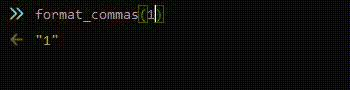Take me back!

# How to add Commas in Numbers

I had to add commas to a number in Javascript today. Thought it was kinda interesting, so here is what I came up with:

```function format_commas(amount) {
let amount_mod = String(amount);
//iterate the amount of commas there are
for (let i=0; i < Math.floor((String(amount).length-1)/3); i++) {
let position = amount_mod.length-3*(i+1)-i;
amount_mod = amount_mod.substring(0, position)+","+amount_mod.substring(position, amount_mod.length);
}
return amount_mod;
}
```

I think the most interesting part of this code was the 5th line (`let position = amount_mod.length-3*(i+1)-i;`). You might be wondering with the `-i` at the end is necessary. That's there because we are increasing the string's length by adding a comma, so we need to offset it.Here is a version that can handle decimals, `undefined` and `NaN`:

```function format_commas(amount) {
if (isNaN(Number(amount))) {
return amount;
}
let before_dec = String(amount).split('.');
let amount_mod = before_dec;
//iterate the amount of commas there are
for (let i=0; i < Math.floor((before_dec.length-1)/3); i++) {
let position = amount_mod.length-3*(i+1)-i;
amount_mod = amount_mod.substring(0, position)+","+amount_mod.substring(position, amount_mod.length);
}
if (String(amount).split('.')) {
amount_mod = amount_mod+"."+String(amount).split('.');
}
return amount_mod;
}
```